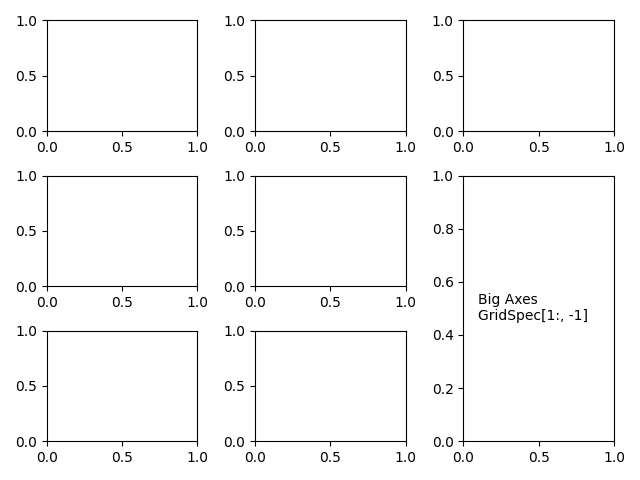# Combining two subplots using subplots and GridSpec#

Sometimes we want to combine two subplots in an axes layout created with subplots. We can get the GridSpec from the axes and then remove the covered axes and fill the gap with a new bigger axes. Here we create a layout with the bottom two axes in the last column combined.

To start with this layout (rather than removing the overlapping axes) use subplot_mosaic.import matplotlib.pyplot as plt

fig, axs = plt.subplots(ncols=3, nrows=3)
gs = axs[1, 2].get_gridspec()
# remove the underlying axes
for ax in axs[1:, -1]:
ax.remove()